^
You are here: HomeFAQs → The Common Core FAQ

# Frequently Asked Questions: the alignment of Math Mammoth to the Common Core Standards (CCS)

This document explains some common questions and answers concerning the common core alignment of Math Mammoth Light Blue series (the complete curriculum), and the changes between the new (revised) and old versions.

1. Which Math Mammoth materials are aligned to the CCS, and which are not?

2. Does Math Mammoth align to the California Common Core Standards?

3. What are the differences between the old version and the new aligned version?

4a. The changes in the common core edition in grade 1

4b. The changes in the common core edition in grade 2

4c. The changes in the common core edition in grade 3

4d. The changes in the common core edition in grade 4

• The 2020 edition in grade 4
• 4e. The changes in the common core edition in grade 5

• The 2020 edition in grade 5
• 4f. The changes in the common core edition in grade 6

5. Is one more advanced (introducing concepts earlier) than the other?

6. Why have you aligned Math Mammoth to the Common Core?

7. What is your position on the Common Core Standards?

8. Does it "dumb down" the books?

9. Can I continue from non-aligned version grade X to aligned version grade X+1?

10. Can I purchase the old, non-aligned versions?

1. Which Math Mammoth materials are aligned to the CCS, and which are not?

Math Mammoth Light Blue series (the complete curriculum for grades 1-7(a)) meets and exceeds the CCS. In several grade levels (grade 3, 5, 6, and 7), the alignment is only 90-95%, because some topics are included that exceed or are not mentioned in the CCS.

Keep in mind this has not been just an alignment but also a revision process. I just cannot look at my old work without finding ways to make it better. So, there have also been improvements in the layout, images, presentation of topics, more word problems, and other changes – independent of the Common Core Standards.

The old version of the Light Blue series is not aligned, and it is available as a download of all 6 grades at this link: Add to cart (\$136 USD).

The Blue Series, Green Series, and Golden Series books are not aligned to the Common Core. The Golden Series books are aligned to the Virginia Standards. Make It Real Learning workbooks are not aligned.

Also, the units I sell at my Teachers Pay Teachers storefront are aligned to the CCS.

2. Does Math Mammoth align to the California Common Core Standards?

California amended the generic Common Core Standards document with THREE additions.

• The first addition is to the standard 2.MD.7 in 2nd grade:

Know relationships of time (e.g., minutes in an hour, days in a month, weeks in a year).

Math Mammoth grade 2 covers the number of days in each month, the thought that there are 60 minutes in an hour, and has extensive practice with dates and calendar, which helps cement the fact that there are seven days in the week. The curriculum does not cover in 2nd grade how many weeks there are in a year. Students continue to work with various time units in both 3rd and 4th grade in Math Mammoth.

4.G.2 Classify two-dimensional figures based on the presence or absence of parallel or perpendicular lines, or the presence or absence of angles of a specified size. Recognize right triangles as a category, and identify right triangles. (Two-dimensional shapes should include special triangles, e.g., equilateral, isosceles, scalene, and special quadrilaterals, e.g., rhombus, square, rectangle, parallelogram, trapezoid.) CA

Math Mammoth covers right, acute, and obtuse triangles in 4th grade, and equilateral, isosceles, and scalene triangles in 5th. The curriculum does cover all the special quadrilaterals listed above, in 4th grade (and in more detail in 5th).

5.OA.2.1 Express a whole number in the range 2-50 as a product of its prime factors. For example, find the prime factors of 24 and express 24 as 2 × 2 × 2 × 3. CA

This is covered both in MM5 and MM6.

3. What are the differences between the old pre-common core version and the new aligned version?

For Math Mammoth Light Blue Series, the alignment has involved moving some topics up a grade level, a few topics down a grade level, adding a few topics, and revising the presentation of some. I haven't actually removed any topics from the curriculum because of this.

In some grade levels, I have left topics in the curriculum that are beyond the CCS, such as some easy conversions between measuring units in grade 3 or many statistics topics in grade 5. Grade 6 will have a review of decimal arithmetic (not mentioned in the CCS). Grade 7 will have the Pythagorean Theorem (in grade 8 in the CCS).

Also, CCS are not all-comprehensive. They are silent about some "lesser" topics such as line graphs, Roman numerals, or learning about parts of the circle. I haven't dropped such topics from Math Mammoth if they were there already. In some topics, CCS are very vague. For example, for one particular grade they say "students work with time and money". Obviously time (clock/calendar) and money are still included in Math Mammoth, just as they were before.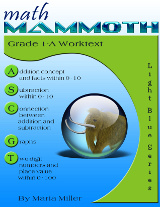4a. What are the differences between the pre-common core and the common core edition in grade 1?

Please note: some of the changes listed below were not due to the Common Core Standards, but were part of a general revision process.

• What used to be chapter 4 (Place Value) switched places with Chapter 3 (Addition and Subtraction Facts within 0-18).
• The topics of rounding, even & odd numbers, and parenthesis were taken off and moved to grades 2 and 3.
• There are many new lessons about adding and subtracting within 20, and adding and subtracting two-digit numbers without regrouping, explaining basic mental math principles.
•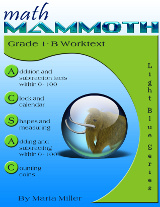The lesson "Exploring Measuring" is split into two lessons (Measuring Length and Exploring Measuring). Both lessons are expanded from what they were before.
• Geometry section was expanded and changed. It now has more material about shapes.

See also: Math Mammoth Common Core alignment, Grade 1 - a list of lessons and the corresponding Common Core Standards in Math Mammoth grade 1 (a PDF file)

4b. What are the differences between the pre-common core and the common core edition in grade 2?

Please note: some of the changes listed below were not due to the Common Core Standards, but were part of a general revision process.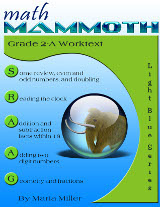• The topics of rounding and finding 1/4 of a number were taken off (moved to grade 3).
• The topics of regrouping in addition and regrouping in subtraction are now split so that 2-A contains regrouping in addition, and 2-B contains regrouping in subtraction.
• There is now more explicit (more scaffolded) instruction about adding four single-digit numbers, and then adding four two-digit numbers in columns (in 2-A).
• Regrouping in subtraction with three-digit numbers is also scaffolded more (the progression of the concept presentation is by smaller steps) (in 2-B). Regrouping with zero tens is still left for third grade, as before (such as in 506 − 358).
• There are more word problems in general. As before the change, the curriculum still has lots of instruction about mental math in addition and subtraction.
•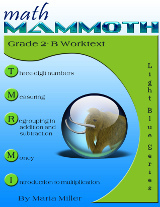Geometry chapter concentrates on naming basic shapes such as triangles, quadrilaterals, pentagons, and hexagons, putting shapes together to form new ones, dividing shapes into new ones, and geometric patterns. The topics of right angle and parallel lines were taken off as they are not in Common Core Standards for grade 2.
• In measuring, the topic of volume is moved to third grade in accordance to Common Core Standards. The topics in 2nd grade include inches, half-inches, centimeters, feet, miles, meters, kilometers, and weight in pounds and in kilograms. Compared to the earlier edition, I have added line plots, exercises about measuring objects both in inches and in centimeters, estimating lengths, and finding how much longer one thing is than another.
• The topics of clock, place value with 3-digit numbers, graphs, and introduction to multiplication didn't have any significant conceptual changes (just cosmetic ones).

See also: Math Mammoth Common Core alignment, Grade 2 - a list of lessons and the corresponding Common Core Standards in Math Mammoth grade 2 (a PDF file)

4c. What are the differences between the pre-common core and the common core edition in grade 3?

Please note: some of the changes listed below were not done because of the Common Core Standards, but because of a general revising process.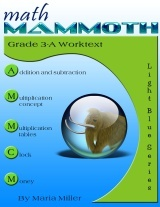What is the same?

• The focus on single-digit multiplication and division is the same. I did add more varied word problems to these two sections, and other little 'enhancements' as I edited the lessons, but no major changes here.
• Also, much of the addition, subtraction, and place value topics are the same. There are some slight differences, but not major ones.
• The time and money sections are the same.

What is different?

• The geometry chapter has been pretty much redone. Now, it concentrates on the concepts of area and perimeter (according to Common Core standards). Before, I did have one lesson on area, but also other concepts, such as right angles and parallel lines. Those will be moved to 4th grade.
•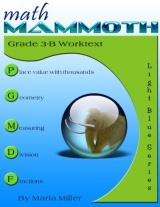In measuring, I have moved most of the content that dealt with conversions between the measuring units to 4th grade. I did leave some content and easy exercises about it, though, as I feel 3rd graders are able to do such. I also reorganized some of the material here and added new measuring activities.
• In fractions, I added the topics of fractions on a number line, equivalent fractions, and comparing fractions, and removed the topic of adding and subtracting like fractions.
• The topic of decimal number has been taken off from this grade.

## 2018-2019 editions

During these years, the lessons on elapsed time were revised.

See also: Math Mammoth Common Core alignment, Grade 3 - a list of lessons and the corresponding Common Core Standards in Math Mammoth grade 3 (a PDF file)

4d. What are the differences between the pre-common core and the common core edition in grade 4?

Please note: some of the changes listed below were not due to the Common Core Standards, but were part of a general revision process.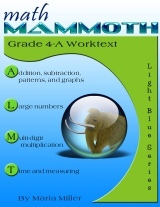The main changes in the revised edition of Grade 4 are that some topics were taken off and moved to 5th grade, and several topics were added in. Other than that, the basic framework and conceptual development did not change substantially. Of course, I edited the lessons to make them better, added more space or grids for calculations, etc. so there were many cosmetic changes.

• Numbers beyond 1,000,000
• multiplying a 3-digit number by a 2-digit number
• 2-digit divisor in long division
• multiplying decimals by whole numbers
• circles
• volume
• In measuring, the problems about conversions between units are easier, and the more challenging conversions will be in 5th grade.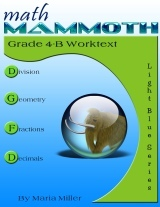• divisibility
• prime numbers
• finding factors of a given number
• multiplying fractions by whole numbers
• adding a fraction with tenths and another with hundredths (such as 3/10 + 8/100)
• Line symmetry

## 2018-2019 editions

During these years, the lessons on elapsed time and some lessons in the geometry chapter on angles were revised.

## The 2020 edition of grade 4

The main differences between the pre-2020 editions of grade 4 and the 2020 and later editions are as follows:

• Several lessons were completely revised (on the topics of order of operations, multiplying money amounts, adding fractions, mixed numbers, subtracting mixed numbers, using decimals with measuring units).
• Some topics are now covered in two lessons instead of one and the lessons were revised (multiplying in parts, the remainder, review of area of rectangles).
• The lesson "Finding Fractional Parts with Division" was moved into a different place within the chapter.
• There are minor changes in individual exercises and text throughout.

See also: Math Mammoth Common Core alignment, Grade 4 - a list of lessons and the corresponding Common Core Standards in Math Mammoth grade 4 (a PDF file)

4e. What are the differences between the pre-common core and the common core edition in grade 5?

Please note: some of the changes listed below were not due to the Common Core Standards, but were part of a general revision process.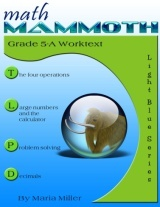The biggest changes in grade 5 are that the two optional chapters (percent and integers) have been removed, and the topics in geometry chapter are different. However, overall, the structure and contents of this grade is very similar to the old. Grade 5 in Math Mammoth slightly exceeds the Common Core Standards.

• Chapter 1 starts out like the old version, with some review topics and multi-digit multiplication. There is now more focus and practice problems on long division with two-digit divisors. One big change is that the lessons on problem solving and equations were moved to their own chapter (chapter 3 in the revised version). Another is that I have now included the topics of divisibility, factors and primes, and prime factorization (used to be in the old version grade 6).
• Chapter 2 (large numbers) is also very similar to the old version. The lesson A Little Bit of Millions was added (it used to be in 4th grade).
• Chapter 3 is about problem solving and simple equations (originally these lessons were in the 1st chapter). This chapter exceeds the CCS.
• The chapter about decimals is very similar to the old version in its contents. The topic of writing decimals in expanded form was added to one of the lessons. Other changes are cosmetic - improved layout, images, scaffolding of concepts, and order of presentation (in some lessons).
• The chapter on graphing and statistics is very similar to the old version. I added one more lesson on patterns in the coordinate grid. This chapter is now Chapter 5, and is moved to the part B.

The chapter exceeds Common Core standards for 5th grade (the lessons on line graphs, histograms, analyzing graphs, mean, and mode), because the CCS do not include statistics topics in 5th grade. However, I feel students will need this as a background before the statistics topics of 6th grade, which are somewhat advanced. For example, in 6th grade students will study interquartile range and/or mean absolute deviation, and will need to relate the choice of measures of center and variability to the shape of the data distribution and the context in which the data were gathered. I do not feel that you can read Common Core Standards as "include this and nothing else", because the standards are not all-inclusive: there are many topics they do not explicitly mention. In my opinion, for students to be able to make sense of mean absolute deviation and relate the choice of measures of center & variability to the shape of the data distribution in 6th grade, they need to analyze and study statistical graphs in easier manners in 5th grade.
•• Chapter 6 is fraction addition and subtraction. It is, again, very similar to the earlier version in its contents. I have added a few new problems about how to spot calculation errors using "fraction number sensen" and about line plots.
• Chapter 7 is fraction multiplication and division. It is similar to the earlier version, but has some changes as well. The lesson Fraction Multiplication and Area is expanded to include problems where the student extends the sides of the given rectangle to get a square unit, and then write a multiplication for the area of the original rectangle. There are two new lesson Multiplication as Scaling/Resizing and Fractions are Divisions. Line plots are included in several of the lessons.

Division of fractions is only dealt in these special cases: sharing divisions (such as (4/6) ÷ 2), dividing unit fractions by whole numbers (for example (1/5) ÷ 3) and dividing whole numbers by unit fractions (such as 5 ÷ (1/3)). These types of divisions can be solved with mental reasoning, without using the "rule." The rest is left for 6th grade.

• Chapter 8 is geometry, and its topics are different from the older version. Now, it includes a review of angles, a review of area and perimeter, drawing circles, classifying triangles, classifying quadrilaterals, and volume of rectangular prisms. It used to include many lessons about the area of polygons, which will now move to 6th grade.

## The 2020 edition of grade 5

The 2020 edition brings some significant changes to Math Mammoth grade 5. The main differences between the pre-2020 editions and the 2020 and later editions are as follows:

• The lessons on the calculator have been completely redone.
• The long chapter on decimals was split into two, and the chapter on graphing and statistics was placed in between. The latter chapter on decimals reviews some of the topics from the first. All the lessons about decimals were revised, and many were completely rewritten to have better scaffolding of the concepts.
• Several lessons on other topics (pan balance and equations , histograms, multiply fractions by whole numbers ) were completely revised.
• Some topics are now covered in two lessons instead of one and the lessons were completely revised (adding and subtracting mixed numbers, multiplying fractions by fractions, volume of rectangular prisms).
• Division of fractions is now approached differently. There is a new lesson about the shortcut for fraction division, and the existing lessons on fraction division were completely redone.
• The topic of ratios (covered in one lesson) was removed from this grade (it is covered in 6th and 7th).
• There are minor changes in individual exercises and text throughout.

See also: Math Mammoth Common Core alignment, Grade 5 - a list of lessons and the corresponding Common Core Standards in Math Mammoth grade 5 (a PDF file)

4f. What are the differences between the pre-common core and the common core edition in grade 6?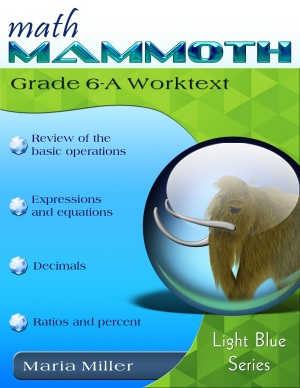In grade 6, there are several substantial changes. In Part A, the first chapter (review of the four operations) is similar to the old, but the rest of 6th grade has lots of changes.

Part A now has more coverage of algebraic concepts (evaluating and simplifying expressions, solving equations, inequalities) than before (chapter 2). The chapter on decimal arithmetic is fairly similar to the old version (chapter 3), but the topics of scientific notation and repeated decimals are moved to 7th. There is now more emphasis on ratios and rates (chapter 4). Percent lessons (chapter 5) are pretty much the same as before, but the topics of interest and percent of change are moved to 7th grade.

In part B, chapter 6 is about number theory, and is similar to the old version, but has a more detailed coverage of the greatest common factor and the least common multiple. In fractions (chapter 7), there is less review of old topics, and more emphasis on division of fractions and lessons on problem solving. Integers chapter is similar (chapter 8), but will omit multiplication and division of integers. Geometry topics (chapter 9) are changing nearly completely and will now emphasize area of polygons and surface area & nets.

Chapter 10 is statistics. It contains many of the same lessons and problems as the old, but has some new emphases, such as: students find the shape of distribution and determine which measure of center and measure of variability is the best to use based on the distribution. The topic of probability is moved to 7th.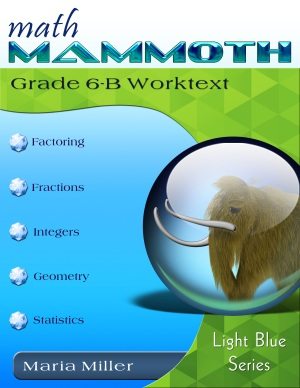• area of triangles and of polygons
• volume of rectangular prisms with fractional edge lengths
• writing, evaluating, and simplifying expressions
• solving simple linear equations
• inequalities
• a more detailed coverage of GCF and LCM
• a more detailed coverage of ratios and rates
• statistics: shape of distributions, mean absolute deviation

Topics moving to 7th grade that used to be in 6th in the old version:

• proportions
• scientific notation and repeated decimals
• percent of change and interest
• multiplication and division of integers
• most geometry topics
• probability

See also: Math Mammoth Common Core alignment, Grade 6 - a list of lessons and the corresponding Common Core Standards in Math Mammoth grade 6 (a PDF file)

Math Mammoth Common Core alignment, Grade 7 - a list of lessons and the corresponding Common Core Standards in Math Mammoth grade 7 (a PDF file)

5. Is one more advanced (introducing concepts earlier) than the other?

For grades 5-6, the old version is more advanced and the CCS aligned version will be less so. However, the old version of MM 6 has been almost "too advanced" as it had many pre-algebra concepts in it. I will be writing a true pre-algebra program (MM7) and move many things from current MM6 to it.

I cannot exactly say one is more advanced than the other for grades 1-4. Some topics moved up a grade (two-digit divisor in long division, even/odd, rounding), but some moved down a grade (perimeter/area of rectangles in depth, factoring). Most topics didn't actually move, because Math Mammoth fit the common core standards in many aspects already, before this revision process. Maybe overall the new, revised version is a tad easier since there have been more concepts that moved up a grade than those that moved down.

6. Why have you aligned Math Mammoth to the Common Core?

I became aware of these standards maybe in 2009 or 2010, but didn't pay any mind to them until late 2011, when I noticed that practically all states had decided to adopt them. So, I took a closer look then. I noticed the standards didn't look bad, AND that Math Mammoth (Light Blue Series) actually was "aligned" in many aspects already, especially in grades 1-5.

Now that I've studied them in detail, I have found a few that are "out of place", but for the most part, I feel the standards are a VAST improvement over most of the states math standards, many of which were really long "laundry lists" that have caused the typical math curricula to be "inch deep and mile long".

I have always felt the long lists of standards was the wrong way to go, as it causes teachers and curricula to teach many, many multitudes of topics with shallowness. The CCS have fewer standards per grade, allowing more focus on certain topics and themes, thus hopefully allowing for true mastery, instead of teachers hurrying through the topics and passing kids to the next grade without them mastering the math.

Also, the common core standards for math are quite similar to how I think math should be taught (and how the old version of MM was written): they emphasize conceptual development a lot, and include the standards algorithms and fact mastery. Like I said, Math Mammoth was already "aligned" in many major aspects to the CCS.

Now, in 2013-2014 there has risen a lot of controversy about the CCS and some states have decided to not to adopt them, for example because of difficulty/cost of implementation and other reasons—and I can understand those! However, I did not know or foresee any of that in 2011 when I saw the map of nearly all states having adopted them. To me, they are just another set of standards, just like the various state standards used to be, with the difference that this time it was possible for me to align the curriculum.

Some have asked me to "revert" back to the old version because of the controversies. However, I also REVISED the curriculum at the same time and spent a LOT of time with various improvements, so I cannot easily just go back to the old. I don't want to "lose" the improvements made in the revising process.

I COULD, technically speaking, say, swap the topic of even numbers back to first grade, and then say "It is not aligned," but to me, that would be silly! Already, grades 3 and 5 are NOT perfectly aligned to the CCS (exceeding the CCS), and grades 6 and 7 will not be. In essence, overall Math Mammoth is maybe 95% aligned to the CCS.

The alignment is definitely good enough that those homeschooling parents who are planning to put their child back to public school in a certain grade and those who need to take yearly tests based on the CCS can be assured that their child has studied the necessary topics. Hopefully I am able to help the homeschooling community in that respect.

7. What is your position on the Common Core Standards?

The answer to this question is here.

8. Does it "dumb down" the books?

I cannot feel so at all, because the Common Core Standards for mathematics emphasize conceptual understanding a lot, and like mentioned above, Math Mammoth already was "aligned" to them in many aspects. I have not made changes that would make the books "dumber"; I could never do that! Math Mammoth is still committed to delivering "conceptual math," the understanding of "why" math works, and lots of word problems, including challenging ones.

Now, some topics have moved up a grade, and some may feel that means the curriculum has gotten "dumbed" down because of that. I do not feel any of those moves are so critical as to make Math Mammoth a "dumb" program. Your child can still start pre-algebra in grade 7.

To give you examples of topics moving up a grade: rounding moved from grade 2 to grade 3, long division with a two-digit divisor and multiplying 3-digit nubers by 2-digit numbers moved from grade 4 to grade 5, and integers & percent moved from grade 5 (they had been optional in MM5 anyway) to grades 6 and 7.

And, some topics have moved down a grade: most notably, factoring and primes to grade 4, and area & perimeter to grade 3. Additionally, grade 6 now has lessons about some algebraic concepts (distributive property, simplifying expressions, inequalities, solving one-step, and some two-step equations). As it is, grade 5 exceeds the CCS somewhat (please see above), and the same will be true of grades 6 and 7.

Also, the presentation of topics has not changed towards "dumber" at all. I wouldn't want such, and I don't know if I could even accomplish such!

9. Can I continue from the non-aligned version in some grade to the aligned version in the next grade?

In general, yes, you can. However, please check the points above as to what was changed in any particular grade level. In some cases the student might need to study a topic before going on with the new, revised version. For example, a child going from old version grade 3 to the new version in grade 4 would need to study area & perimeter of rectangles in depth as it is now covered in grade 3. You can also email me for advice.

10. Can I purchase the old, non-aligned versions?

The old versions are also available as printed books at Lulu. They're titled "original" version.

However, since I have improved upon my older work in the revised versions, I don't feel the old versions are as good as the new.

WAIT!

Receive my monthly collection of math tips & resources directly in your inbox — and get a FREE Math Mammoth book!You can unsubscribe at any time.

### Math Mammoth Tour

Confused about the different options? Take a virtual email tour around Math Mammoth! You'll receive:

An initial email to download your GIFT of over 400 free worksheets and sample pages from my books. Six other "TOURSTOP" emails that explain the important things and commonly asked questions concerning Math Mammoth curriculum. (Find out the differences between all these different-colored series!)

This way, you'll have time to digest the information over one or two weeks, plus an opportunity to ask me personally about the curriculum.
A monthly collection of math teaching tips & Math Mammoth updates (unsubscribe any time)### "Mini" Math Teaching Course

This is a little "virtual" 2-week course, where you will receive emails on important topics on teaching math, including:

- How to help a student who is behind
- Troubles with word problems
- Teaching multiplication tables
- Why fractions are so difficult
- The value of mistakes
- Should you use timed tests
- And more!

A GIFT of over 400 free worksheets and sample pages from my books right in the very beginning.A monthly collection of math teaching tips & Math Mammoth updates (unsubscribe any time)
Enter your email to receive math teaching tips, resources, Math Mammoth news & sales, humor, and more! I tend to send out these tips about once monthly, near the beginning of the month, but occasionally you may hear from me twice per month (and sometimes less often).• A GIFT of over 400 free worksheets and sample pages from my books.